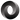## 测试KaTeX数学公式

Tue, 6th August 2019Edit on Githubjavascriptes6

\begin{alignedat}{2} 10&x+ &3&y = 2 \\ 3&x+&13&y = 4 \end{alignedat} $\def\arraystretch{1.5} \begin{array}{c:c:c} a & b & c \\ \hline d & e & f \\ \hdashline g & h & i \end{array}$

test change from copy of local machine.

mathe 来测试一下: $e^{i\pi} +1 =0$

A brief introduction about various uses of var, let, const .

Github